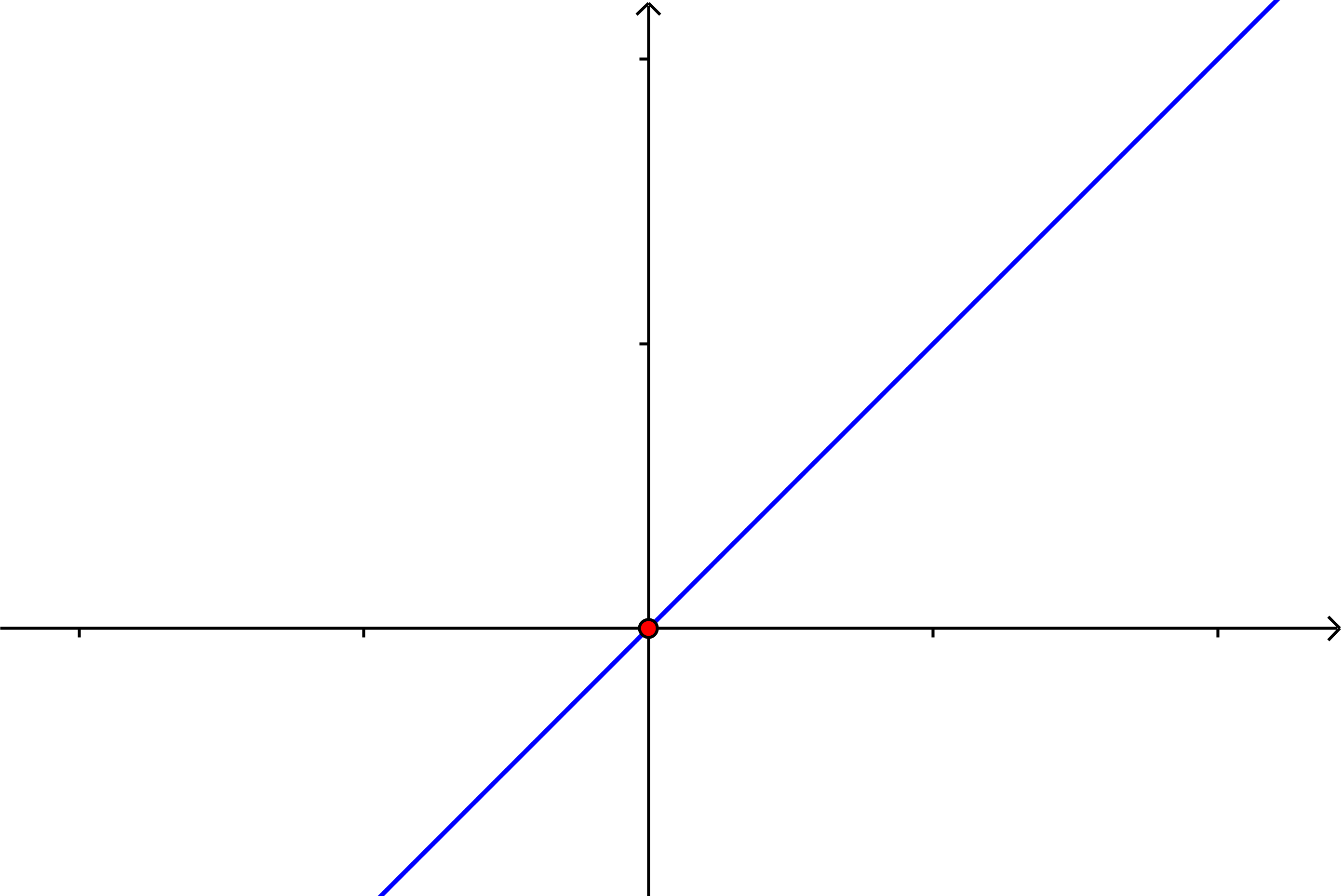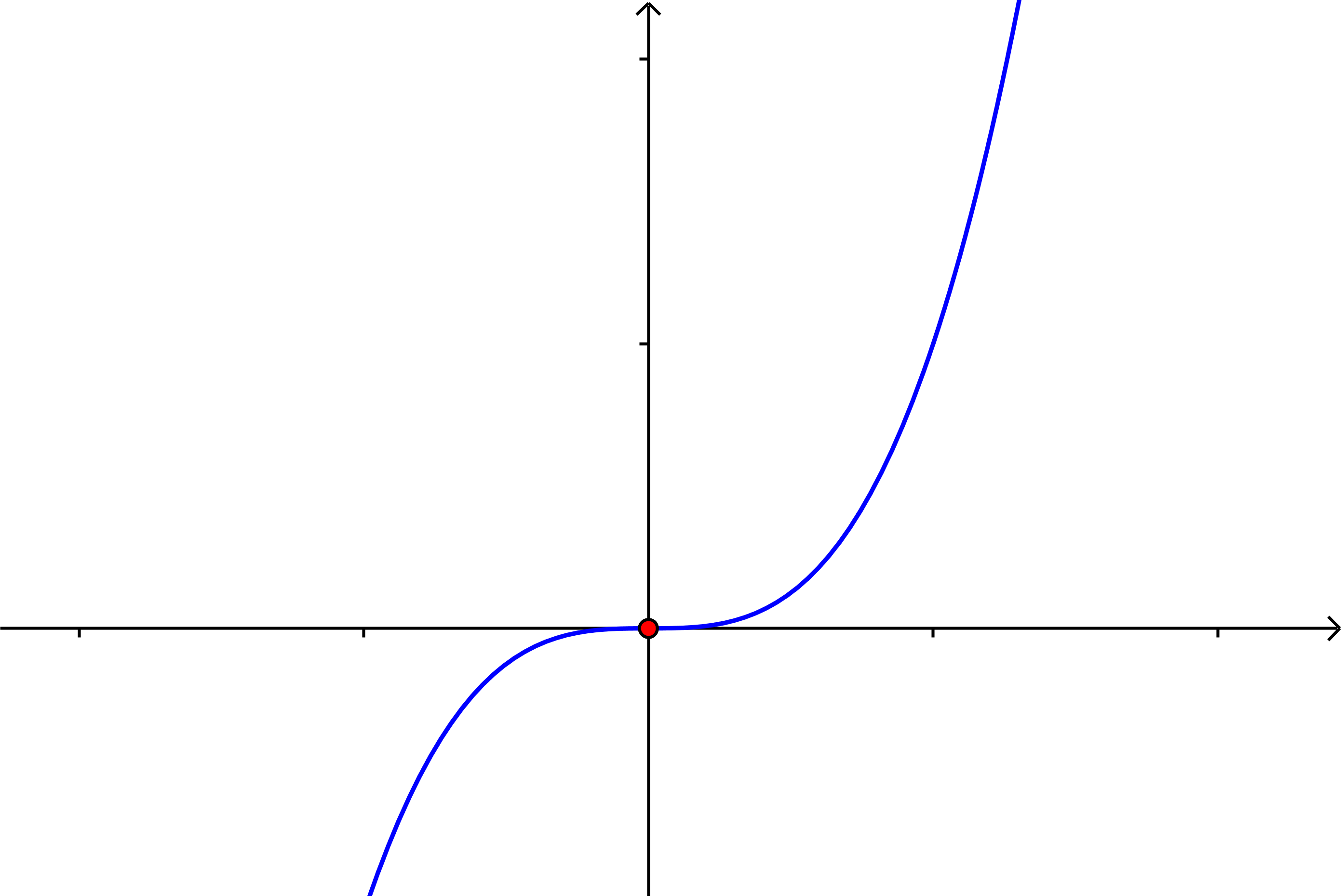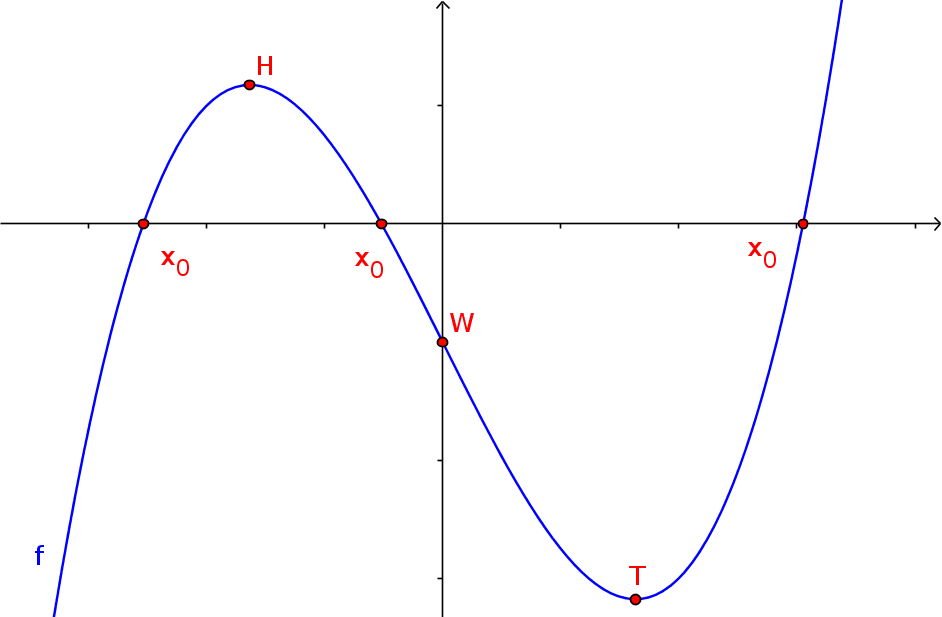Math Real functions Polynomial functions

# Polynomial functions

Polynomial functions have an equation in the form:

$f(x)=a_n\cdot x^n+a_{n-1}\cdot x^{n-1}+...$ $+a_1\cdot x+a_0$
i

### Hint

Polynomial functions are a special type of rational functions $\frac{g(x)}{h(x)}$ with the denominator $h(x) = 1$.

The function term is called polynomial and the exponent $n$ indicates the degree of the polynomial.

The following are typical function graphs for nth degree functions:

 $n=1$ linear functions* $f(x)=x$$n=2$ quadratic functions* $f(x)=x^2$$n=3$ cubic functions* $f(x)=x^3$* In the examples, all functions are also power functions, but linear, quadratic and cubic functions are only power functions if there is no other summand behind them. For example, these are not power functions: $f(x)=x+5$ and $f(x)=4x^2-x+3$

!

### Note

Power functions of the form $f(x)=ax^n$ are only polynomial functions if $n\in\mathbb{N}$.
Power functions with $n\in\mathbb{Z}$ and $n<0$ (e.g. $x^{-3}$) are rational functions.

### Examples

Other examples of polynomial functions are:

• $f(x)=10x-5$

• $f(x)=x^3+x$

• $f(x)=x^4+10x^3+2x^2+4$
!

### Remember

The graph of an nth degree function has at most

• $n$ zeros
• $(n-1)$ extrema
• $(n-2)$ inflection points

### Example

The pictured 3rd degree function has:
• three zeros ($x_0$)
• two extrema ($H$ = maximum and $T$ = minimum)
• one inflection point ($W$)